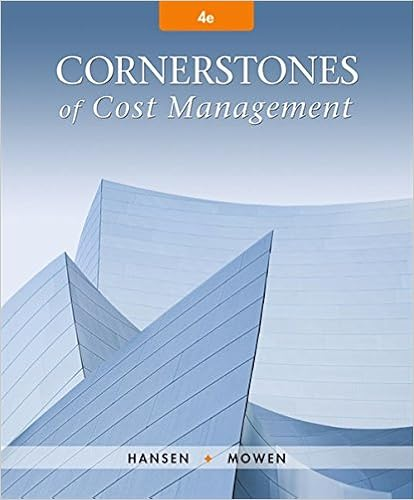# A 2 and 5 b 1 and 6 c 2 and 6 d 4 and 5 110 consider

• Homework Help
• 101
• 97% (65) 63 out of 65 people found this document helpful

This preview shows page 66 - 69 out of 101 pages.

##### We have textbook solutions for you!
The document you are viewing contains questions related to this textbook.The document you are viewing contains questions related to this textbook.
Chapter 4 / Exercise 1
Cornerstones of Cost Management
Hansen/MowenExpert Verified
A.2 and 5B. 1 and 6C. 2 and 6D. 4 and 5
##### We have textbook solutions for you!
The document you are viewing contains questions related to this textbook.The document you are viewing contains questions related to this textbook.
Chapter 4 / Exercise 1
Cornerstones of Cost Management
Hansen/MowenExpert Verified
110. Consider the following three product costing alternatives: process costing, job order costing, and standard costing. Which of these can be used in conjunction with absorption costing? A. job order costingB. standard costingC. process costingD.all of the above111. Another name for absorption costing is A.full costing.B. direct costing.C. job order costing.D. fixed costing.112. If a firm produces more units than it sells, absorption costing, relative to variable costing, will result in A.higher income and assets.B. higher income but lower assets.C. lower income but higher assets.D. lower income and assets.113. Under absorption costing, fixed manufacturing overhead could be found in all of the following exceptthe A. work-in-process account.B. finished goods inventory account.C. Cost of Goods Sold.D.period costs.114. If a firm uses absorption costing, fixed manufacturing overhead will be included A. only on the balance sheet.B. only on the income statement.C.on both the balance sheet and income statement.D. on neither the balance sheet nor income statement.115. Under absorption costing, if sales remain constant from period 1 to period 2, the company will report a larger income in period 2 when A.period 2 production exceeds period 1 production.B. period 1 production exceeds period 2 production.C. variable production costs are larger in period 2 than period 1.D. fixed production costs are larger in period 2 than period 1.67| P a g e
116. The FASB requires which of the following to be used in preparation of external financial statements? A. variable costingB. standard costingC. activity-based costingD.absorption costing117. An ending inventory valuation on an absorption costing balance sheet would A. sometimes be less than the ending inventory valuation under variable costing.B. always be less than the ending inventory valuation under variable costing.C. always be the same as the ending inventory valuation under variable costing.D.always be greater than or equal to the ending inventory valuation under variable costing.118. Absorption costing differs from variable costing in all of the following exceptA. treatment of fixed manufacturing overhead.B.treatment of variable production costs.C. acceptability for external reporting.D. arrangement of the income statement.119. Which of the following isnotassociated with absorption costing? A. functional formatB. gross marginC. period costsD.contribution margin120. Unabsorbed fixed overhead costs in an absorption costing system are A.fixed manufacturing costs not allocated to units produced.B. variable overhead costs not allocated to units produced.C. excess variable overhead costs.D. costs that cannot be controlled.121. Profit under absorption costing may differ from profit determined under variable costing. How is this difference calculated?
•••